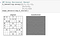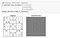# Image Filters with Convolutions

## Using Python’s scipy library for implementation

In this post, I will discuss about Convolutions and how they act as image filters by implementing convolution operation using a few edge detection kernels.

So let’s start!

## What are Convolutions?

Convolutions are mathematical operation on two functions that produces a third function that expresses how one is modified by the other.

To give an example, the first function can be the image and the second function is a matrix sliding over the image(kernel) that results in transforming the input image. Kernel works by multiplying the patch of the image corresponding to the kernel’s size and summing up the result obtained and then sliding over the image to perform the same process again.

Thus, Convolution operation can help in extracting features from images with the help of kernels. For more details on Convolutions and Kernels, please refer to my previous blog here.

## Image Filters with Convolutions

In order to demonstrate how convolution operation can be used as Image Filters, I have used signal.convolve2d function from the scipy library of Python that outputs convolution result for two 2D arrays.

• Importing required libraries
• Defining a function to detect features from the image using specified kernel
• Detecting horizontal edgesTransformed image after convolution operation using a horizontal edge detector kernel
• Detecting vertical edgesTransformed image after convolution operation using a vertical edge detector kernel
• Detecting diagonal edges